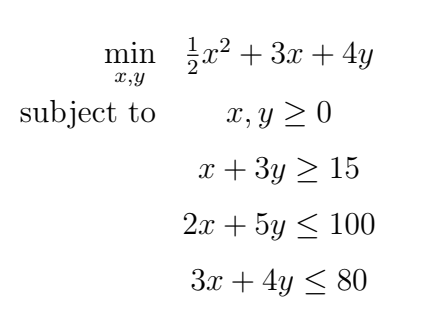## Saturday, May 18, 2019

Note that xT denotes the transpose of x, and Ax < b means that the inequality is taken element-wise over the vectors Ax and b. The above objective function is convex if and only if H is positive- semidefinite. The quadprog function expects a problem of the above form, defined by the parameters fH; f; A; b; Aeq; beq; l; u; x0; H and f are required, the others are optional (empty matrix [])

General Formula
Examplerewrite pattern above

Code in matlab

clc;clear all;close all;
H = diag([1; 0]);
f = [3; 4];
A = [-1 -3; 2 5; 3 4];
b = [-15; 100; 80];
l = zeros(2,1);
Aeq = [];
Beq = [];
u = [];
x0 = [];
options = optimset('Algorithm','interior-point-convex');



Output

>> x

x =

0.0000
5.0000

>> fval

fval =

20.0000

>>


We can verify3 that x = 0; y = 5, with an optimal value 20.